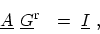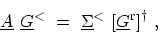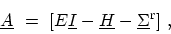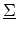# H. Recursive GREEN's Function Method

As discussed in Section 4.2, the matrix representation of the kinetic equations is given by(H.1)(H.2)(H.3)

whereis the total self-energy. To calculate the GREEN's functions, matrix inversion is required. However, since the matrices are block tridiagonal and also most of the observables are related to the diagonal blocks of the GREEN's functions, one can employ a recursive method to calculate the observables efficiently [116,8]. We follow here the method proposed in .
Subsections M. Pourfath: Numerical Study of Quantum Transport in Carbon Nanotube-Based Transistors